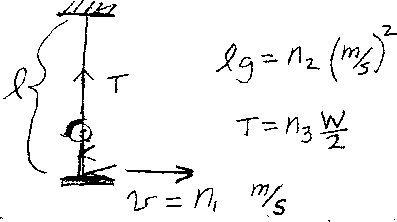Problem B12: George is swinging on a playground swing. The length of the swing is l, and George's weight is W. At the bottom of the swing, George's speed is v=n1 meters/sec. The value of l times g is equal to lg = n2 (meters/sec)2. If the swing is held up by two ropes, what is the tension T in each rope. That is, if T = n3 W/2, what is n3? Note, that if the speed were zero, the tension in each rope would be W/2.n1 = n2 = Input n3:
If you are currently in my class, you can record your grade by entering your name and student ID number (without the leading zeros) below and clicking on "record grade".
 First Name = Last Name = ID = Problem: